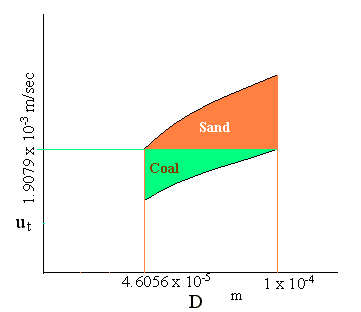### Screening And Classification

A mixture of coal and sand particles having sizes smaller than 1 x 10-4 m in diameter is to be separated by screening and subsequent elutriation with water. Recommend a screen aperture such that the oversize from the screen can be separated completely into sand and coal particles by elutriation. Calculate also the required water velocity. Assume that Stokes law is applicable. Density of sand = 2650 kg/m3; density of coal = 1350 kg/m3; density of water = 1000 kg/m3; viscosity of water = 1 x 10-3 kg/m.s; g = 9.812 m/s2.

Calculations:

For laminar settling regimes, terminal-settling velocity is given by

Stokes law: (valid for NRe,p < 0.1)

ut = D2s - ρ)g / (18m)

where D is the diameter of particle

ρs is the density of solid

where, NRe,p = Dutρ/μ

ut of larger dia coal particle = (1 x 10-4 )2 x (1350 - 1000) x 9.812 / (18 x 0.001)

= 1.9079 x 10-3 m/sec

Dia of sand particle corresponding to the ut of larger dia coal particle:

1.9079 x 10-3 = D2 x (2650 - 1000) x 9.812 / (18 x 0.001)

D = 4.6056 x 10-5 m

The size of screen aperture needed so that the oversize particles can be separated completely into sand and coal by elutriation = 4.6056 x 10-5 m

The required water velocity = 1.9079 x 10-3 m/sec

By operating at the water velocity of 1.9079 x 10-3 m/sec, coal particles will be carried along with water, and sand particles will settle down.

This calculation is aided with the following diagram: